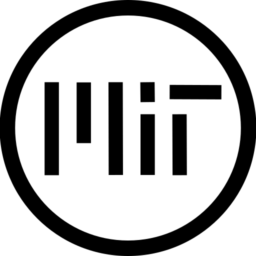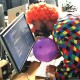# CMYK Halftone

A canvas item shader that attempts to emulate the look of halftone printing, like something you would see in a newspaper. It is capable of both object space and screen space shading. Some compromises where made since it’s real time rendering, so it’s not completely accurate. Feel free to improve.

Notes and how-tos:

• An example of a texture for the Halftone Dither Pattern is included as one of the screenshots in this post. It also need to have repeat enabled in import settings to work.
• If object_space is false it uses screen space.
• You can see what most of the parameters do by simply dragging the sliders.
• Might be slow at high pattern_scaling and resolution, try lowering sampling_quality if it’s slow.
• Have ‘filter’ turned on in image import settings. Pixel art will look good filtered with this shader.

References:

``````shader_type canvas_item;
// KNOWN ISSUES:
// - Unfiltered images create sharp unnatural transitions. Filtered pixel art looks fine here. Don't worry.
// - Result is a bit too saturated.
// - Animate offsets slightly with TIME.
// - Paper texture.
// - Distance maps for transparent images.

/****** TO USE object_space TWO TRANSFORMS HAS TO BE FED TO THE SHADER IN process() ******/
// The canvasitem-inherited node needs this script:
/*
#tool # If you want to see the shader in the editor.
extends CanvasItem

func _process(_delta):
*/
/**** NOTE: The script above isn't needed in screen space mode ****/

uniform float sampling_quality: hint_range(0.0, 1.0) = 1.0;

uniform float pattern_scaling: hint_range(1.05, 100.0) = 20.0;

uniform float cyan_pattern_rotation: hint_range(-180, 180) = 0.0;
uniform float magenta_pattern_rotation: hint_range(-180, 180) = 15.0;
uniform float yellow_pattern_rotation: hint_range(-180, 180) = 30.0;
uniform float black_pattern_rotation: hint_range(-180, 180) = 45.0;

uniform float alpha_threshold: hint_range(0.0, 1.0) = 0.5;

uniform vec4 paper_color: hint_color = vec4(1.0);

uniform vec4 cyan_color: hint_color = vec4(0.0, 1.0, 1.0, 1.0);
uniform vec4 magenta_color: hint_color = vec4(1.0, 0.0, 1.0, 1.0);
uniform vec4 yellow_color: hint_color = vec4(1.0, 1.0, 0.0, 1.0);
uniform vec4 black_color: hint_color = vec4(0.0, 0.0, 0.0, 1.0);

uniform vec2 cyan_offset_position = vec2(0.0,0.0);
uniform vec2 magenta_offset_position = vec2(0.0,0.0);
uniform vec2 yellow_offset_position = vec2(0.0,0.0);
uniform vec2 black_offset_position = vec2(0.0,0.0);

uniform float cyan_offset_rotation: hint_range(-180.0, 180.0) = 0.0;
uniform float magenta_offset_rotation: hint_range(-180.0, 180.0) = 0.0;
uniform float yellow_offset_rotation: hint_range(-180.0, 180.0) = 0.0;
uniform float black_offset_rotation: hint_range(-180.0, 180.0) = 0.0;

uniform bool object_space = false;
uniform mat4 canvas_global_transform;
uniform mat4 camera_global_transform;

// MUST HAVE REPEAT ENABLED
uniform sampler2D halftone_dither_pattern;

varying vec2 world_p;

// https://matthew-brett.github.io/teaching/rotation_2d.html
vec2 rotated(vec2 v, float d_angle) {
return vec2(x,y);
}

// tl_uv, tr_uv, bl_uv, br_uv: top left corner, top right corner etc. l_uv: uv within those four corners.
vec4 one_pass(vec2 tl_uv, vec2 tr_uv, vec2 bl_uv, vec2 br_uv, vec2 l_uv, sampler2D tex) {
vec2 top_uv = mix(tl_uv, tr_uv, l_uv.x);
vec2 bot_uv = mix(bl_uv, br_uv, l_uv.x);
vec2 pass_uv = mix(top_uv, bot_uv, l_uv.y);

// Offset the base color UV for each CMYK channel
vec2 c_uv = rotated(pass_uv + cyan_offset_position, cyan_offset_rotation);
vec2 m_uv = rotated(pass_uv + magenta_offset_position, magenta_offset_rotation);
vec2 y_uv = rotated(pass_uv + yellow_offset_position, yellow_offset_rotation);
vec2 k_uv = rotated(pass_uv + black_offset_position, black_offset_rotation);

vec4 c_base_color = texture(tex, c_uv);
vec4 m_base_color = texture(tex, m_uv);
vec4 y_base_color = texture(tex, y_uv);
vec4 k_base_color = texture(tex, k_uv);

// Get base_colors' CMYK values. Source: https://www.rapidtables.com/convert/color/rgb-to-cmyk.html
float c_k = 1.0 - max(max(c_base_color.r, c_base_color.g), max(c_base_color.g, c_base_color.b));
float m_k = 1.0 - max(max(m_base_color.r, m_base_color.g), max(m_base_color.g, m_base_color.b));
float y_k = 1.0 - max(max(y_base_color.r, y_base_color.g), max(y_base_color.g, y_base_color.b));

float k = 1.0 - max(max(k_base_color.r, k_base_color.g), max(k_base_color.g, k_base_color.b));
float c = (1.0 - c_base_color.r - c_k) / (1.0 - c_k);
float m = (1.0 - m_base_color.g - m_k) / (1.0 - m_k);
float y = (1.0 - y_base_color.b - y_k) / (1.0 - y_k);
float a = texture(tex, pass_uv).a < alpha_threshold ? 0.0f : 1.0f;

ivec2 pattern_size = textureSize(halftone_dither_pattern, 0);
vec2 screen_size = vec2(textureSize(tex, 0));
vec2 inv_pattern_size = vec2(1.0 / float(pattern_size.x), 1.0 / float(pattern_size.y));
vec2 pattern_uv = pass_uv * inv_pattern_size * screen_size * pattern_scaling;
float threshold = texture(halftone_dither_pattern, pattern_uv).r;
// A threshold for each CMYK channel. And each one has differently rotated pattern_uv
vec2 c_pattern_uv = rotated(pattern_uv, cyan_pattern_rotation);
vec2 m_pattern_uv = rotated(pattern_uv, magenta_pattern_rotation);
vec2 y_pattern_uv = rotated(pattern_uv, yellow_pattern_rotation);
vec2 k_pattern_uv = rotated(pattern_uv, black_pattern_rotation);

float c_threshold = texture(halftone_dither_pattern, c_pattern_uv).r;
float m_threshold = texture(halftone_dither_pattern, m_pattern_uv).r;
float y_threshold = texture(halftone_dither_pattern, y_pattern_uv).r;
float k_threshold = texture(halftone_dither_pattern, k_pattern_uv).r;

vec3 paper_base = paper_color.rgb;

// Setting UVs outside of [0, 1] to paper color.
if (c > c_threshold && c_uv.x >= 0.0 && c_uv.x <= 1.0 && c_uv.y >= 0.0 && c_uv.y <= 1.0)
{ paper_base = paper_base - ((paper_color.rgb - cyan_color.rgb)); }
if (m > m_threshold && m_uv.x >= 0.0 && m_uv.x <= 1.0 && m_uv.y >= 0.0 && m_uv.y <= 1.0)
{ paper_base = paper_base - ((paper_color.rgb - magenta_color.rgb)); }
if (y > y_threshold  && y_uv.x >= 0.0 && y_uv.x <= 1.0 && y_uv.y >= 0.0 && y_uv.y <= 1.0)
{ paper_base = paper_base - ((paper_color.rgb - yellow_color.rgb)); }
if (k > k_threshold && k_uv.x >= 0.0 && k_uv.x <= 1.0 && k_uv.y >= 0.0 && k_uv.y <= 1.0)
{ paper_base = paper_base - ((paper_color.rgb - black_color.rgb)); }

return vec4(paper_base, a);
}

void vertex() {
world_p = (canvas_global_transform * vec4(VERTEX, 0.0, 1.0)).xy;
}

void fragment() {
vec2 sample_uv;
vec4 sample_color = vec4(0.0);
if (object_space) {
// Object based multi sampling using the canvas_global_transform and camera_global_transform
// Get the UVs of the pixels corners
float camera_x_basis_length = length(vec2(camera_global_transform, -camera_global_transform));
float camera_y_basis_length = length(vec2(-camera_global_transform, camera_global_transform));
vec2 tl_g_pos = world_p + vec2(0.5 / camera_x_basis_length, 0.5 / camera_y_basis_length);
vec2 tr_g_pos = world_p + vec2(0.5 / camera_x_basis_length, -0.5 / camera_y_basis_length);
vec2 bl_g_pos = world_p + vec2(-0.5 / camera_x_basis_length, 0.5 / camera_y_basis_length);
vec2 br_g_pos = world_p + vec2(-0.5 / camera_x_basis_length, -0.5 / camera_y_basis_length);

mat4 inv_canvas = inverse(canvas_global_transform);
vec2 texture_res = vec2(textureSize(TEXTURE,0));
vec2 tl_uv = (inv_canvas * vec4(tl_g_pos, 0.0, 1.0)).xy / texture_res - vec2(-0.5);
vec2 tr_uv = (inv_canvas * vec4(tr_g_pos, 0.0, 1.0)).xy / texture_res - vec2(-0.5);
vec2 bl_uv = (inv_canvas * vec4(bl_g_pos, 0.0, 1.0)).xy / texture_res - vec2(-0.5);
vec2 br_uv = (inv_canvas * vec4(br_g_pos, 0.0, 1.0)).xy / texture_res - vec2(-0.5);

// Dynamic amount of samples based on the amount of CMYK dot pixels that fit within the current fragment.
vec2 p_uv_1 = tl_uv * texture_res;
vec2 p_uv_2 = tr_uv * texture_res;
vec2 p_uv_4 = bl_uv * texture_res;
vec2 p_uv_3 = br_uv * texture_res;
float area_term_1 = ((p_uv_1.x * p_uv_2.y) - (p_uv_2.x * p_uv_1.y));
float area_term_2 = ((p_uv_2.x * p_uv_3.y) - (p_uv_3.x * p_uv_2.y));
float area_term_3 = ((p_uv_3.x * p_uv_4.y) - (p_uv_4.x * p_uv_3.y));
float area_term_4 = ((p_uv_4.x * p_uv_1.y) - (p_uv_1.x * p_uv_4.y));
float pixel_area = abs(area_term_1 + area_term_2 + area_term_3 + area_term_4) / 2.0;
float pixel_area_sqrt = clamp(sqrt(pixel_area), 1.0, 10.0);

int samples = int(ceil(pixel_area_sqrt * pattern_scaling * sampling_quality));
int sqrt_samples = int(ceil(sqrt(float(samples))));
// Sample 'samples' amount of times with even spacing within those pixel corners
samples = sqrt_samples * sqrt_samples;
for (int x = 0; x < sqrt_samples; x++) {
float x_progress = float(x) / (float(sqrt_samples - 1));
for (int y = 0; y < sqrt_samples; y++) {
float y_progress = float(y) / (float(sqrt_samples - 1));
sample_uv = vec2(x_progress, y_progress);
sample_color = sample_color + (one_pass(tl_uv, tr_uv, bl_uv, br_uv, sample_uv, TEXTURE)
/ float(samples));
}
}
}
else {
// This is screen space multi sampling
// Get the SCREEN_UVs of the pixels corners
vec2 tl_s_uv = (FRAGCOORD.xy + vec2(0.5, 0.5)) * SCREEN_PIXEL_SIZE;
vec2 tr_s_uv = (FRAGCOORD.xy + vec2(0.5, -0.5)) * SCREEN_PIXEL_SIZE;
vec2 bl_s_uv = (FRAGCOORD.xy + vec2(-0.5, 0.5)) * SCREEN_PIXEL_SIZE;
vec2 br_s_uv = (FRAGCOORD.xy + vec2(-0.5, -0.5)) * SCREEN_PIXEL_SIZE;
int samples = int(ceil(pattern_scaling * sampling_quality));
int sqrt_samples = int(ceil(sqrt(float(samples))));
// Sample 'samples' amount of times with even spacing within those pixel corners
samples = sqrt_samples * sqrt_samples;
for (int x = 0; x < sqrt_samples; x++) {
float x_progress = float(x) / (float(sqrt_samples - 1));
for (int y = 0; y < sqrt_samples; y++) {
float y_progress = float(y) / (float(sqrt_samples - 1));
sample_uv = vec2(x_progress, y_progress);
sample_color = sample_color + (one_pass(tl_s_uv, tr_s_uv, bl_s_uv, br_s_uv, sample_uv, SCREEN_TEXTURE)
/ float(samples));
}
}
}
COLOR = sample_color;
}``````
###### Tags
dither, HalftoneThe shader code and all code snippets in this post are under MIT license and can be used freely. Images and videos, and assets depicted in those, do not fall under this license. For more info, see our License terms.

### Halftone

Subscribe
Notify ofInline Feedbackskrnr
1 year ago

I was looking into recreating Mrmo Tarius’ shader today – perfect timing 🙂

I get all white when attempting to apply it to a sprite. Could you perhaps provide an example project?krnr
1 year ago

I did. Still no dice 🙁krnr
1 year ago